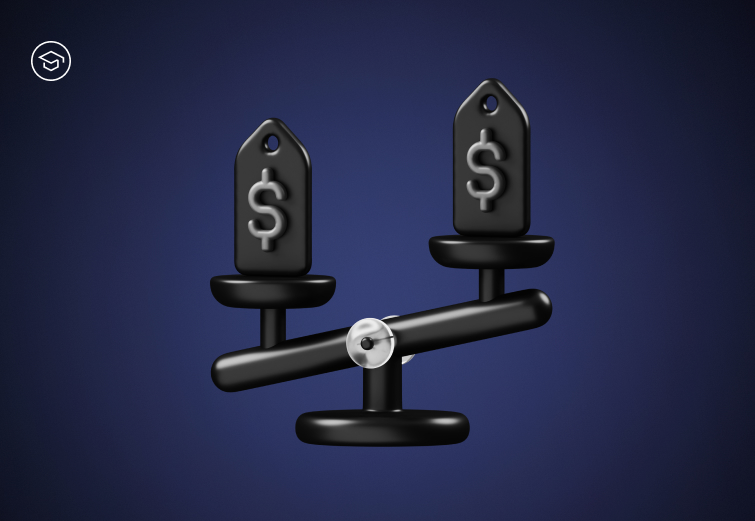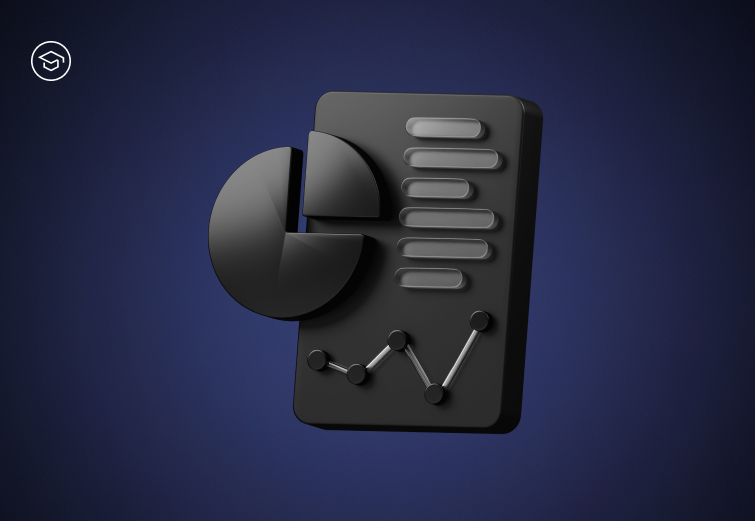STC Educational Panel

## Introduction to the Bollinger Bands

March 28, 2023
9mThe Bollinger Bands are a technical indicator used in financial analysis. They identify various elements affecting the asset's price, such as periods when the price is considered low or high compared to its recorded volatility.

#### How do they work?

To understand the mechanism of the Bollinger Bands, it is necessary to explain their structure and discover the correlations between the various bands.

The indicator has three lines indicating a specific asset's price volatility range.

The first line (middle band) is the moving price average calculated based on a designated number of periods. This average determines the trend direction and provides a reference point for the other lines in the bands.

The second line (top line) is calculated by adding a standard deviation of the price to the moving average value. By default, this standard deviation value is 2, meaning the ribbon's top and bottom lines are two points away from the middle band.

The third line (bottom line) is obtained by subtracting the standard deviation of the price from the value of the moving average.

#### How to calculate the Bollinger Bands?

The formula to calculate the Bollinger Bands is complex, but it can be summarized in the following steps:

1. Calculate the Simple Moving Average (SMA) of the closing prices for a given period. The most commonly used period is 20 days, but traders may use others, depending on their preferences and the characteristics of the analyzed asset.

Remember that SMA is calculated as an arithmetic average of the closing prices over a specified period.

2.
Calculate the standard deviation of the closing prices for the same period used in step 1. The formula takes the sum of the squared deviations and divides by the total number of values in the set. The end value represents the variance of the data, which is then squared rooted to obtain the standard deviation.

The formula for this calculation is as follows:

Standard Deviation = √( Σ (x - μ)² / N )
where:
x – individual data value
μ – mean or average value
N = total number of data values

Let’s assume we want to calculate the standard deviation for “X” with five values – 5, 7, 8, 10 and 12.

Firstly, calculate the mean: (5 + 7 + 8 + 10 + 12) / 5 = 8.4

Then, for each value, calculate the deviation from the mean, and square each of them:
(5 - 8.4) = -3.4 —> (-3.4)² = 11.56
(7 - 8.4) = -1.4 —> (-1.4)² = 1.96
(8 - 8.4) = -0.4 —> (-0.4)² = 0.16
(10 - 8.4) = 1.6 —> (1.6)² = 2.56
(12 - 8.4) = 3.6 —> (3.6)² = 12.96

Then, calculate the average of the squared deviations:
(11.56 + 1.96 + 0.16 + 2.56 + 12.96) / 5 = 5.44

Lastly, take the square root of the result from step 4:
√5.44 = 2.33

Therefore, the standard deviation of this set is
2.33.

Calculate the top and bottom bands by adding and subtracting a number of standard deviations from the SMA. The most commonly used number is 2, but traders may use others depending on preferences and assets.

The formulas for calculating Top and Bottom of Bollinger Bands are as follows:

Top Bollinger Band = SMA x [(High+Low+Close Price)/3n, n] + mσ [TP, n]

Bottom Bollinger Band = SMA x [(High+Low+Close Price)/3n, n] − mσ [TP,n]

where:
SMA – Simple Moving Average
n – number of days in the smoothing period – usually 20-period
m – number of standard deviations, usually 2-period
σ[TP,n]=Standard Deviation over last n periods of
(High+Low+Close) Price

#### Identification of market trends

The Middle Band may be utilized to determine the trend. If an asset's price stays above the middle band, the asset is in an uptrend, and if it stays below the middle band, it's in a downtrend.

#### Determining support and resistance levels

The Bottom Bollinger Band defines the support level, and the Top Band represents the resistance level.

When the current price crosses the bottom line, it may mean the price is undervalued, and you may consider opening a long position. Still, if it crosses the top line, the price may be overvalued, which may suggest opening a short position.

#### Identification of periods of variability

When the price of an asset approaches the Bottom Band, this can be a signal to buy the asset.

Conversely, this may be a sign to consider selling when the price comes to the Top Band.

#### Determining the degree of volatility

The distance between the Top and Bottom Bollinger bands indicates the degree of volatility of the asset. The wider the bands, the higher the volatility.

#### Summary

The Bollinger Bands is a popular technical analysis indicator that consists of three lines marking the moving average and the upper and lower limits of price volatility.

Their use makes it possible to determine the trend direction, support, resistance levels, and periods of high and low volatility, allowing traders to decide on entering or exiting positions.

Complete quiz
Introduction to the Bollinger BandsIntermediate

### Spoofing

Spoofing is a technique that involves falsifying information, such as IP or e-mail addresses, phone numbers, or login details, to deceive a system or user and gain unauthorized access. Spoofing can be employed in various attacks, including phishing, DDoS, or man-in-the-middle.Intermediate

### Volume-Weighted Average Price (VWAP)

VWAP is the volume-weighted average price of trades that took place in a given time frame. It is used by traders to analyze the current market price and compare it to the value of the weighted average.Beginner

### Introduction to Fundamental Analysis

Fundamental Analysis (FA) is a method of evaluating the intrinsic value of an asset, which supports investors in making informed financial decisions.